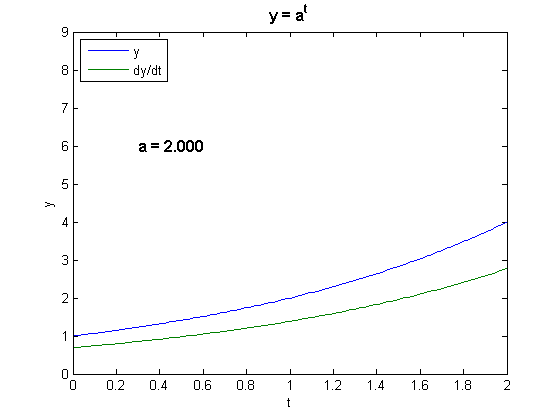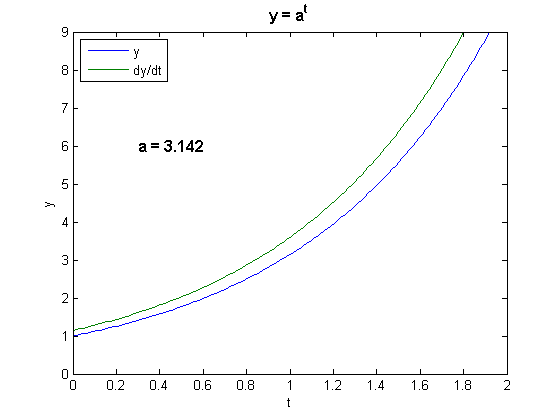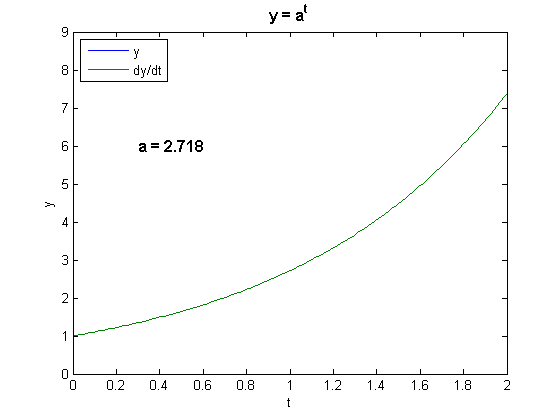# Exponential Growth

What, exactly, is exponential growth? What is e and what does it have to do with exponential growth? A simple MATLAB interactive graphic introduces these concepts.

### Contents

#### Exponential growth.

To most people "exponential growth" simply means "very rapid growth". But, more precisely, a time varying quantity grows expontially if the rate of growth is proportional to size of the quantity itself. The rate can even be negative, in which case it is "exponential decay". I think that students who have taken calculus in high school or college should understand the mathematical ideas involved in exponential growth, but I'm afraid that most of them don't. When I ask students to tell me the derivative of $t^3$, they can usually respond $3t^2$. When I ask them "why?", they say "take the $3$, put it out in front, and subtract $1$ from the exponent". Finding derivatives is a purely mechanical process, like adding fractions or solving quadratic equations. When I ask for the derivative of $3^t$, some will even apply the same process to get $t 3^{t-1}$. There is no understanding of the relationship between differentiation and rate of change. A function $f(t)$ is growing exponentially if its growth rate, its derivative, is proportional to the function itself. Perhaps the most important function in all of mathematics is the one where this proportionality constant is equal to one, so the function is its own derivative. Let's discover that function.

#### Approximate derivative.

We can get numerical values and graphs of derivatives without actually differentiating anything. For our purposes, approximate derivatives based on the notion of rate of change are, in some ways, even preferable to actual derivatives. We just have to pick a small step size $h$, say $h = .0001$. Then the approximate derivative of $f(t)$ is $$\dot{f}(t) = \frac{f(t+h)-f(t)}{h}$$

#### 2^t

What do we mean by the function $$f(t) = 2^t$$ If $t$ is a positive integer, then $2^t$ is $2$ multiplied by itself $t$ times. $$2^0 = 1, \ \ 2^1 = 2, \ \ 2^2 = 4, ...$$ If $t$ is a negative integer, then $2^t$ is $1/2$ multiplied by itself $|t|$ times. $$2^{-1} = 1/2, \ \ 2^{-2} = 1/4, ...$$ If $t = p/q$ is a rational number, the ratio of two integers, $2^{p/q}$ is the $q$-th root of the $p$-th power of $2$. $$2^{1/2} = \sqrt{2} = 1.4142, \ \ 2^{355/113} = \sqrt{2^{355}} = 8.8250, ...$$ Theoretically, for floating point arithmetic, this is all we need to know. All floating point numbers are ratios of two integers. We do not have to be concerned yet about the definition of $2^t$ for irrational $t$. If MATLAB can compute powers and roots, we can plot the graph of $2^t$.

#### Interactive interface.

The function expgui is included with the software for the book Experiments with MATLAB. I invite you to download the function and run it. It plots the graph of $a^t$ and its approximate derivative. Here is the code that generates the initial plot, with $a = 2$. You can see that the derivative, in green, has the same shape as the function, in blue. This is exponential growth.
   t = 0:1/64:2;
h = .0001;

% Compute y = a^t and its approximate derivative

a = 2.0;
y = a.^t;
ydot = (a.^(t+h) - a.^t)/h;

% Plot

plot(t,[y; ydot])

% Label

axis([0 2 0 9])
fs = get(0,'defaulttextfontsize')+2;
text(0.3,6.0,'a = 2.000','fontsize',fs,'fontweight','bold')
title('y = a^t','fontsize',fs,'fontweight','bold')
legend('y','dy/dt','location','northwest')
xlabel('t')
ylabel('y')#### Animation.

At this point, if you are actually running expgui, you can move the blue line with your mouse, changing the value of $a$. If you don't have MATLAB, or haven't downloaded expgui, you can click on this movie to see a simulation of the animation. I hope you get to move the line yourself with expgui. The tactile experience is much more satisfying that just watching the movie.

#### pi^t

In case you are not able to run expgui or watch the movie, here is the plot of $\pi^t$ and its approximate derivative.
   a = pi;
y = a.^t;
ydot = (a.^(t+h) - a.^t)/h;
p = get(gca,'children');
set(p(3),'ydata',y)
set(p(2),'ydata',ydot)
set(p(1),'string','a = 3.142')#### Finding e.

You should soon see that the graph of the derivative of $a^t$ always has the same shape as the graph of $a^t$ itself. If $a$ is less than $2.7$ the derivative is below the function, while if $a$ is greater than $2.8$ the derivative is above the function. By moving the mouse carefully you can find a value in between where the curves lie on top of each other, The critical value of $a$ is 2.718. You have discovered $e$ and $e^t$, the only function in the world that is equal to its own derivative. And, you didn't have to differentiate anything. Here is the final graph.
   y = exp(t);
p = get(gca,'children');
set(p(3),'ydata',y)
set(p(2),'ydata',y)
set(p(1),'string','a = 2.718')#### e^t

In contrast to its equally famous cousin, $\pi$, the actual numerical value of $e$ is not so important. It's the function $e^t$, or exp(t) as it's known in MATLAB, that is fundamental. If you ever need to know the value of $e$, you can always use
format long
e = exp(1)

e =

2.718281828459046


It's pretty easy to memorize the first ten significant figures.
fprintf('e = %12.9f\n',e)

e =  2.718281828


Published with MATLAB® 7.14

|